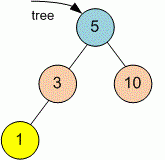Competitions

# Tree Minimum depth

Given a binary tree, find its minimum depth. The minimum depth is the number of nodes along the shortest path from the root node down to the nearest leaf node.

Definition of a tree:

// Java
class TreeNode
{
public:
int val;
TreeNode left;
TreeNode right;
TreeNode(int x) {
val = x;
left = NULL;
right = NULL;
};

// C++
class TreeNode
{
public:
int val;
TreeNode *left;
TreeNode *right;
TreeNode(int x) : val(x), left(NULL), right(NULL) {}
};


Implement function minDepth that returns the minimum depth of the tree.

// Java
int minDepth(TreeNode tree)

// C++
int minDepth(TreeNode *tree)


#### ExampleFunction minDepth returns 2 because the shortest path from the root 5 to the nearest leaf 10 contains 2 nodes.

Time limit 1 second
Memory limit 128 MiB
Author Mykhailo Medvediev